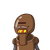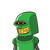# A man bought some pens for ₹60. If the price of each pen had been ₹1 less, he would have got 3 pens more, how much did each pen co

A man bought some pens for ₹60. If the price of each pen had been ₹1 less, he would have got 3 pens more, how much did each pen cost him (in ₹)​

### 2 thoughts on “A man bought some pens for ₹60. If the price of each pen had been ₹1 less, he would have got 3 pens more, how much did each pen co”

1.A boy bought some pencils for \$60. If he had paid \$1 less for each pencil, he could have bought 5 more pencils. How many pencils did he pay for?

Now calculation starts as,

As per the information given , if we decrease the cost per pencil by 1 then we can get more of 5 pencils, so

Taking a simple approach

We get a hint that it might be a number nearing 5, that is a cost for one pencil, so

60/5=12

And reducing amount per pencil by one,

60/4=15

Similarly ,

60/3=20

i.e, +5 than the previous number of pencils bought.

And volla, we have the answer is 15.

2.Rs 5

Step-by-step explanation:

Cost of pencils = Total Cost / Cost of 1

Cost of pencils = 60/x

Cost of pencils with Rs 1 less = 60/(x-1)

So, [60/(x-1)] – [60/x] = 3

=> 60x – 60x + 60 = 3x² – 3x

=> 3x² – 3x- 60=0

=> x² – x – 20 = 0

=> x² – 5x + 4x – 20 = 0

=> x(x – 5) + 4(x – 5) = 0

=> (x + 4)(x – 5) = 0

x=5 as x cannot be negative . Thus Rs5 is the cost of each pencil and for Rs60 initially 60÷5 = 12 pencils could have been got.